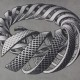# Lesson 27 Solution

Exercise 1) Find 3 non-trivial subgroups of the group$\{0, 1, 2, 3, 4, 5, 6, 7, 8, 9, 10, 11\}$ with addition modulo 12.

Solution: To be non-trivial, our subgroups cannot be the subset$\{0\}$, nor can they be the whole group.  It turns out there are four non-trivial subgroups, so even though I’ve only asked for three (for some unknown reason), I’ll provide all four here.  They are:

1)$\{0, 2, 4, 6, 8, 10\}$,

2)$\{0, 3, 6, 9\}$,

3)$\{0, 4, 8\}$

4)$\{0, 6\}$.

All of these subsets have the three properties of being closed under addition, containing the identity, and containing all inverses.  For example, in 2), the inverse of$6$ is$6$ and the inverse of$9$ is$3$.  I encourage the reader to check that all of the other subgroups satisfy all of these requirements, and then also to explore subgroups of other sets with modular arithmetic defined on them.

Back to the lesson

On to Lesson 28

### 3 Responses to Lesson 27 Solution

1.yoel halb says:

•yoel halb says:
•TrueBeautyOfMath says: